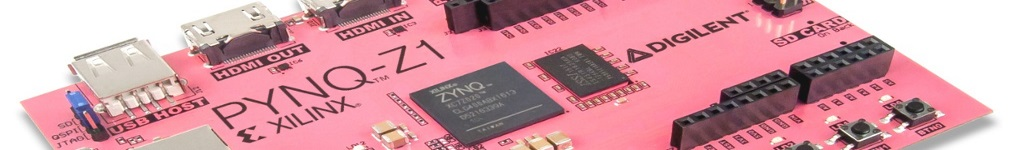PYNQ: PYTHON PRODUCTIVITY FOR ZYNQFail to transfer data between zynq and block memory using cdma

I tried to use `CDMA` to transfer zynq’s data into block memory and after doing some operation(multiplication) and then retrieve answer from block memory to `zynq`.
Last, I can read answer in `zynq` memory through `mmio`.

Following is ip what I use

I expect to write input(a) in address : 0x30000000 and input(b) in :0x30000004 and output(ans) in
0x3000000C

this is my jupyter code, are there anything wrong in my code?

``````     from pynq import Overlay
design = Overlay('./new.bit')
design.ip_dict

sys_in1 = 0x30000000 # zynq's addr

from pynq import MMIO

sys = MMIO(sys_in1,0x14)
ctrl = MMIO(cdma_ctrl,0x18)
sa = MMIO(cdma_sa,0x8)
da = MMIO(cdma_da,0x8)
btt = MMIO(cdma_btt,0x10)

sys.write(0x0,6)  # a
sys.write(0x4,6)  # b

ctrl.write(0x0,0x04)
btt.write(0x0,0x8)

ctrl.write(0x0,0x04)
btt.write(0x0,0x10)

``````

following is verilog code for multiplication

``````    module mul16(rst, clk, R_req, addr, R_data, W_req, W_data);

input 			rst;
input 			clk;
output			R_req;
input	[31:0]	R_data;
output	[3:0]	W_req;
output	[31:0]	W_data;

wire w_r;

reg		[1:0]	C_state;
wire	[1:0]	N_state;

reg 	[31:0]	indata	[1:0];

assign W_data = indata[15:0] * indata[15:0];
assign N_state = C_state + 1;
assign w_r = C_state & C_state;
assign R_req = 1;
assign W_req = {w_r, w_r, w_r, w_r};

always@(posedge clk or negedge rst)begin

if(!rst)begin
C_state <= 0;
indata <= 0;
indata <= 0;

end

else begin
C_state <= N_state;
indata[N_state]<=R_data;
end

end

endmodule``````

Hi,
You don’t need the DMA for what you are currently doing.

You could use MMIO to write directly to the BRAM from the ARM PS.

The DMA is for streaming larger amounts of data, rather than single data reads/writes.

I didn’t check the DMA and if you are using it in the right way/writing data to the write addresses, and I only skimmed your RTL, I’m not sure if it is correct. Looks like you cycle through addresses 0x0, 0x4, 0x8, 0xc and ignoring 0x8 and relying on the timing of your design to effectively ignore the propagation of the input from 0x8. Did you check the timing of the data coming from the BRAM (simulate), and the timing reports for your design? I think it is OK, but this may be causing a problem.

For information, in your code, you can memory map the full register space for an IP with a single command.
e.g.

``````dma_mmio = MMIO(cdma_address, 0xffff)
``````

where 0xffff is the max size of the register address space.

You can access all the registers from their offsets:

``````dma_mmio.read(cdma_sa)
dma_mmio.write(cdma_btt, 0xff)
``````

In your code, you have this mapping,

You need to write “offset” 0x0 for each write.

``````ctrl.write(0x0,0x04)
``````dma.write(CTRL_REG,0x04)